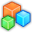# Microsoft Small BasicProgram Listing: JJG240-0
nFig = 1
If nFig = 1 Then
Else
EndIf
width = ImageList.GetWidthOfImage(blackImage)
height = ImageList.GetHeightOfImage(blackImage)
GraphicsWindow.Width = 2*width
GraphicsWindow.Height = nFig*height
GraphicsWindow.DrawImage(blackImage,0,0)
'GraphicsWindow.DrawImage(whiteImage,0,height)

For fig = 1 To nFig ' Process the bacack and white images in turn
red = ""
green = ""
blue = ""
For i = 0 To width-1
For j = (fig-1)*height To fig*height-1
pixel = GraphicsWindow.GetPixel(i,j)

'Get the RGB componentent and concert to decimals 0 - 255
hex = Text.GetSubText(pixel,2,2)
hex2dec()
r = dec
hex = Text.GetSubText(pixel,4,2)
hex2dec()
g = dec
hex = Text.GetSubText(pixel,6,2)
hex2dec()
b = dec

'Count the total number of each vale for reporting
red[r] = red[r]+1
green[g] = green[g]+1
blue[b] = blue[b]+1

'Perhaps the black and white images could be combined nicely to get a different image
If (fig = 1) Then
'Black image - modify and draw pixels not exactly black
If (r+g+b <> 0) Then
If (r = 0) Then
r = 255
EndIf
If (g = 0) Then
g = 255
EndIf
If (b = 0) Then
b = 255
EndIf
GraphicsWindow.SetPixel(width+i,j,GraphicsWindow.GetColorFromRGB(r,g,b))
EndIf
Else
'Black image - modify and draw pixels not exactly white
If (r+g+b <> 3*255) Then
If (r = 255) Then
r = 0
EndIf
If (g = 255) Then
g = 0
EndIf
If (b = 255) Then
b = 0
EndIf
GraphicsWindow.SetPixel(width+i,j,GraphicsWindow.GetColorFromRGB(r,g,b))
EndIf
EndIf
EndFor
EndFor
'Report total cound of each RGB values
TextWindow.WriteLine("Red value count for fig "+fig+" : "+red)
TextWindow.WriteLine("Green value count for fig "+fig+" : "+green)
TextWindow.WriteLine("Blue value count for fig "+fig+" : "+blue)
EndFor

Sub hex2dec
'Convert a hex double digit to a decimal
high = Text.GetSubText(hex,1,1)
low = Text.GetSubText(hex,2,1)
If (high+0 <> high) Then
high = Text.GetCharacterCode(high)-55
EndIf
If (low+0 <> low) Then
low = Text.GetCharacterCode(low)-55
EndIf
dec = 16*high+low
EndSub